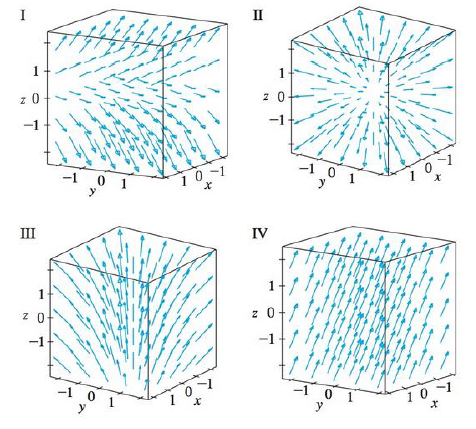Chapter 16.1, Problem 18E

Chapter
Section
Textbook Problem

Match the vector fields F on ℝ3 with the plots labeled I-IV. Give reasons for your choices.18. F(x, y, z) = x i + y j + z kTo determine

To match: The vector field F(x,y,z)=xi+yj+zk with the plots labeled as I-IV.

Explanation

Given data:

F(x,y,z)=xi+yj+zk=x,y,z

Formula used:

Consider a three-dimensional vector F=x,y,z .

Write the expression for length of the three dimensional vector.

|F(x,y,z)|=x2+y2+z2 (1)

Find the length of F(x,y,z) using equation (1)

Still sussing out bartleby?

Check out a sample textbook solution.

See a sample solution

The Solution to Your Study Problems

Bartleby provides explanations to thousands of textbook problems written by our experts, many with advanced degrees!

Get Started

Differentiate f and find the domain of f. f(x) = ln(x2 2x)

Single Variable Calculus: Early Transcendentals, Volume I